Gradient descent with exact line search for a quadratic function of multiple variables

Setup

This page describes gradient descent with exact line search for a quadratic function of multiple variables. Since the function is quadratic, its restriction to any line is quadratic, and therefore the line search on any line can be implemented using Newton's method. Therefore, the analysis on this page also applies to using gradient descent using Newton's method for a quadratic function of multiple variables.

Function

The function we are interested is a function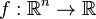$f: \R^n \to \R$ of the form:$f(\vec{x}) = \vec{x}^TA\vec{x} + \vec{b}^T\vec{x} + c$

where$A$ is a$n \times n$ symmetric positive-definite matrix with entries$a_{ij}$ and$\vec{b}$ is the column vector with entries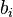$b_i$. For part of this page, we will generalize somewhat to the case that$A$ is a symmetric positive-semidefinite matrix.

Note that we impose the condition of symmetry because we could replace the matrix$A$ with the symmetric matrix$(A + A^T)/2$ without changing the functional form. The positive-definite or positive-semidefinite condition is to guarantee that the function has the right sort of convexity. In the positive-definite case, we are guaranteed that there is a unique point of local minimum that is also the point of absolute minimum. The positive-semidefinite case is somewhat more complicated: we could either have no minimum, or we could have an affine space worth of points at which the function has a local and absolute minimum.

Learning algorithm

The algorithm starts with a guess$\vec{x}_0$ and updates according to the following rule. We use superscripts to denote the iterates, to avoid confusion with the use of subscripts for coordinates.$\vec{x}^{(k+1)} = \vec{x}^{(k)} - \alpha_k \nabla f(\vec{x}^{(k)})$

where$\alpha_k$ is the second derivative of$f$ along the direction of the gradient vector. Explicitly, if we set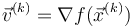$\vec{v}^{(k)} = \nabla f (\vec{x}^{(k)})$, we have: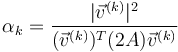$\alpha_k = \frac{|\vec{v}^{(k)}|^2}{(\vec{v}^{(k)})^T(2A)\vec{v}^{(k)}}$

where the matrix$2A$ in the denominator arises as the Hessian of the function$f$. Since the function is quadratic, the Hessian is globally constant.

Convergence properties

We will carry out our analysis of eigenvalues in terms of the Hessian matrix$2A$. If we were instead dealing with the eigenvalues of$A$, we would have to double them to get the eigenvalues of$2A$. Since the bounds on the learning rate are described as reciprocals of the eigenvalues, we would therefore obtain additional factors of 2 in the denominators, or equivalently, we would remove the factor of 2 from the numerators.

Note that in the case$n = 1$, we have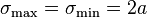$\sigma_{\max} = \sigma_{\min} = 2a$.

Detailed analysis after transforming the problem to a setting where the coordinates are simple

Suppose the eigenvalues of$2A$ are:$\sigma_\max = \sigma_1 \ge \sigma_2 \ge \sigma_3 \ge \dots \ge \sigma_n = \sigma_\min$

Via a coordinate change of the domain using an orthogonal matrix, we can transform$A$ into a diagonal matrix with positive real entries. These entries are halves of the eigenvalues of$2A$. Via a translation, we can get rid of the linear term, and finally, we can translate the function value by a constant (this does not affect the point of local minimum, though it affects the minimum value). We can therefore obtain the following simplified functional form:$f(\vec{x}) = \frac{1}{2} \sum_{i=1}^n \sigma_ix_i^2$

The unique local and absolute minimum is at the zero vector.

Note that even though we know that our matrix can be transformed this way, we do not in general know how to bring it in this form -- if we did, we could directly solve the problem without using gradient descent (this is an alternate solution method). However, even though we may not know the explicit diagonalized form of the function, the fact that it does have such a form gives us information about how the gradient descent process converges.

Starting with a point:$\vec{x} = (x_1,x_2,\dots,x_n)$

we obtain that the gradient vector at the point is:$\nabla f(\vec{x}) = (\sigma_1x_1,\sigma_2x_2,\dots,\sigma_nx_n)$

The multiplier$\alpha$ is: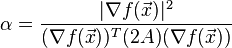$\alpha = \frac{|\nabla f(\vec{x})|^2}{(\nabla f(\vec{x}))^T(2A)(\nabla f(\vec{x}))}$

This simplifies to:$\alpha = \frac{\sum_{j=1}^n \sigma_j^2x_j^2}{\sum_{i=1}^n \sigma_j^3x_j^2}$

Therefore, the update in each coordinate is as follows:$x_i^{\mbox{new}} = x_i - \sigma_i x_i \frac{\sum_{j=1}^n \sigma_j^2x_j^2}{\sum_{i=1}^n \sigma_j^3x_j^2}$

This can also be simplified as: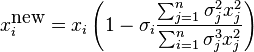$x_i^{\mbox{new}} = x_i\left(1 - \sigma_i \frac{\sum_{j=1}^n \sigma_j^2x_j^2}{\sum_{i=1}^n \sigma_j^3x_j^2} \right)$

The one-dimensional case

In the case that$n = 1$, gradient descent with exact line search converges in one iteration for a quadratic function. In fact, it is equivalent to Newton's method for optimization of a function of one variable, which we know converges in one iteration for a quadratic function.

We can verify this using the above expression: if$n = 1$, then after one iteration, we get to 0, the point of local and absolute minimum.

The two-dimensional case

The two-dimensional case is qualitatively distinct from the general case in the following respect: if we consider the vectors obtained after an even number of iterations, these are collinear and heading towards the optimum at a constant convergence rate. Using the simplified setup here, we work out the details, including the convergence rate.

In our setup, the function has the function: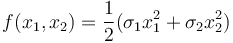$f(x_1,x_2) = \frac{1}{2}(\sigma_1 x_1^2 + \sigma_2x_2^2)$

We have the rule from above:$x_i^{\mbox{new}} = x_i\left(1 - \sigma_i \frac{\sigma_1^2x_1^2 + \sigma_2^2x^2}{\sigma_1^3x_1^2 + \sigma_2^3x_2^2}\right)$

Because there are only two coordinates, the expressions can be simplified somewhat:$x_1^{\mbox{new}} = x_1 \frac{\sigma_2^2 x_2^2(\sigma_2 - \sigma_1)}{\sigma_1^3x_1^2 + \sigma_2^3x_2^2}$

Similarly: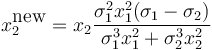$x_2^{\mbox{new}} = x_2 \frac{\sigma_1^2 x_1^2(\sigma_1 - \sigma_2)}{\sigma_1^3x_1^2 + \sigma_2^3x_2^2}$

Suppose we start with the zeroth iterate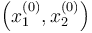$\left(x_1^{(0)}, x_2^{(0)}\right)$. For convenience, we omit superscripts for the zeroth iterate, i.e., we set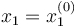$x_1 = x_1^{(0)}$,$x_2 = x_2^{(0)}$. Applying the above gives us:$\mbox{Eq. 1}: x_1^{(1)} = x_1 \frac{\sigma_2^2 x_2^2(\sigma_2 - \sigma_1)}{\sigma_1^3x_1^2 + \sigma_2^3x_2^2}$

Similarly: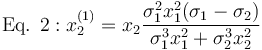$\mbox{Eq. 2}: x_2^{(1)} = x_2 \frac{\sigma_1^2 x_1^2(\sigma_1 - \sigma_2)}{\sigma_1^3x_1^2 + \sigma_2^3x_2^2}$

We also have similar expressions for$x_1^{(2)}, x_2^{(2)}$: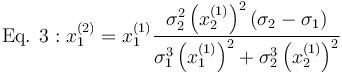$\mbox{Eq. 3}: x_1^{(2)} = x_1^{(1)}\frac{\sigma_2^2 \left(x_2^{(1)}\right)^2(\sigma_2 - \sigma_1)}{\sigma_1^3\left(x_1^{(1)}\right)^2 + \sigma_2^3\left(x_2^{(1)}\right)^2}$$\mbox{Eq. 4}: x_2^{(2)} = x_2^{(1)}\frac{\sigma_1^2 \left(x_1^{(1)}\right)^2(\sigma_1 - \sigma_2)}{\sigma_1^3\left(x_1^{(1)}\right)^2 + \sigma_2^3\left(x_2^{(1)}\right)^2}$

We now want to work out the expression$\sigma_1^3 \left(x_1^{(1)}\right)^2 + \sigma_2^3 \left(x_2^{(1)}\right)^2$, that appear in the denominator for calculating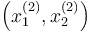$\left(x_1^{(2)}, x_2^{(2)}\right)$. Let's do that. We simply plug in the expressions of$x_1^{(1)}$ and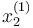$x_2^{(1)}$ from the first two of the above four equations.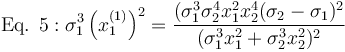$\mbox{Eq. 5}: \sigma_1^3 \left( x_1^{(1)} \right)^2 = \frac{(\sigma_1^3 \sigma_2^4 x_1^2 x_2^4 (\sigma_2 - \sigma_1)^2}{(\sigma_1^3 x_1^2 + \sigma_2^3 x_2^2)^2}$

Similarly: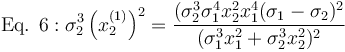$\mbox{Eq. 6}: \sigma_2^3 \left( x_2^{(1)} \right)^2 = \frac{(\sigma_2^3 \sigma_1^4 x_2^2 x_1^4 (\sigma_1 - \sigma_2)^2}{(\sigma_1^3 x_1^2 + \sigma_2^3 x_2^2)^2}$

Note that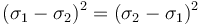$(\sigma_1 - \sigma_2)^2 = (\sigma_2 - \sigma_1)^2$. Adding up, we get: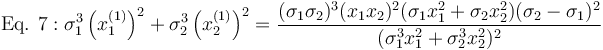$\mbox{Eq. 7}: \sigma_1^3 \left(x_1^{(1)}\right)^2 + \sigma_2^3 \left(x_2^{(1)}\right)^2 = \frac{(\sigma_1 \sigma_2)^3 (x_1x_2)^2 (\sigma_1 x_1^2 + \sigma_2 x_2^2)(\sigma_2 - \sigma_1)^2}{(\sigma_1^3x_1^2 + \sigma_2^3x_2^2)^2}$

Plugging in the results from Equations 1, 2, and 7 into Equations 3 and 4, we obtain:$x_1^{(2)} = x_1 \left(\frac{\sigma_1\sigma_2 (\sigma_2 - \sigma_1)^2(x_1x_2)^2}{(\sigma_1x_1^2 + \sigma_2 x_2^2)(\sigma_1^3x_1^2 + \sigma_2^3x_2^2)}\right)$

Similarly:$x_2^{(2)} = x_2 \left(\frac{\sigma_1\sigma_2 (\sigma_2 - \sigma_1)^2(x_1x_2)^2}{(\sigma_1x_1^2 + \sigma_2 x_2^2)(\sigma_1^3x_1^2 + \sigma_2^3x_2^2)}\right)$

In particular, note that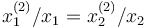$x_1^{(2)}/x_1 = x_2^{(2)}/x_2$, so every two iterations, we move directly in the line toward the minimum.

Moreover, the common value equals: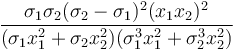$\frac{\sigma_1\sigma_2 (\sigma_2 - \sigma_1)^2(x_1x_2)^2}{(\sigma_1x_1^2 + \sigma_2 x_2^2)(\sigma_1^3x_1^2 + \sigma_2^3x_2^2)}$

Sanity checks:

• If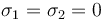$\sigma_1 = \sigma_2 = 0$, convergence is immediate. This makes sense -- we are converging in only one coordinate, and the problem reduces to the one-dimensional case.
• If$\sigma_1 = \sigma_2$, convergence is immediate, because we move coordinated amounts in both directions.

As in the earlier section, assume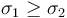$\sigma_1 \ge \sigma_2$, so$\sigma_\max = \sigma_1$ and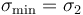$\sigma_\min = \sigma_2$. The value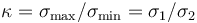$\kappa = \sigma_\max/\sigma_\min = \sigma_1/\sigma_2$ is the condition number. The above expression works out to: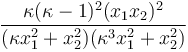$\frac{\kappa (\kappa - 1)^2(x_1x_2)^2}{(\kappa x_1^2 + x_2^2)(\kappa^3 x_1^2 + x_2^2)}$

The linear convergence rate on the domain side is the square root of this quantity, because we get this multiple every two iterations, i.e., the linear convergence rate is:$\sqrt{\frac{\kappa (\kappa - 1)^2(x_1x_2)^2}{(\kappa x_1^2 + x_2^2)(\kappa^3 x_1^2 + x_2^2)}}$

To obtain the linear convergence rate on the function side, we square back, and obtain:$\frac{\kappa (\kappa - 1)^2(x_1x_2)^2}{(\kappa x_1^2 + x_2^2)(\kappa^3 x_1^2 + x_2^2)}$

Note that the convergence rate is sensitive to the choice of starting point. Let's make cases based on starting point:

•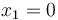$x_1 = 0$: In this case, convergence occurs in one step.
•$x_2 = 0$: In this case, convergence occurs in one step.
• Both$x_1$ and$x_2$ are nonzero. In this case, the convergence rate is positive. Let's compute bounds on this.

Suppose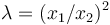$\lambda = (x_1/x_2)^2$. The convergence rate simplifies to:$\frac{\kappa (\kappa - 1)^2\lambda}{(\kappa \lambda + 1)(\kappa^3 \lambda + 1)}$

After doing some calculus to minimize this function, we find that the minimum occurs when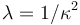$\lambda = 1/\kappa^2$, or equivalently, when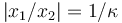$|x_1/x_2| = 1/\kappa$. In other words, the convergence is slowest when the values in the coordinates are inversely proportional to the eigenvalues. In this case, the convergence rate on the domain side works out to the following:$\frac{\kappa - 1}{\kappa + 1}$

The convergence rate on the function value side works out to: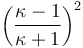$\left(\frac{\kappa - 1}{\kappa + 1} \right)^2$

In this worst-case scenario, the iterates alternate between the lines$x_1/x_2 = \kappa$ and$x_1/x_2 = -\kappa$.

Note that, even in this worst-case scenario, the convergence rate matches the best possible convergence rate we can get for gradient descent (attained when the learning rate is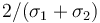$2/(\sigma_1 + \sigma_2)$) as discussed on the page gradient descent with constant learning rate for a quadratic function of multiple variables.